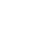## TRENDING :

Python Programmings For Practice Part 1 ( 2022 )### Python program to check whether the given number is even or not.

``````number = https://janakkafle.com/python-programmings-for-practice-part-1-2022#Python_program_to_check_whether_the_given_number_is_even_or_notinput("Enter a number ")
x = int(number)%2
if x == 0:
print(" The number is Even ")
else:
print(" The number is odd ")
``````

### Python program to convert the temperature in degree centigrade to Fahrenheit

``````c = input(" Enter temperature in Centigrade: ")
f = (9*(int(c))/5)+32
print(" Temperature in Fahrenheit is: ", f)
``````

### Python program to find the area of a triangle whose sides are given

``````import math
a = float(input("Enter the length of side a: "))
b = float(input("Enter the length of side b: "))
c = float(input("Enter the length of side c: "))
s = (a+b+c)/2
area = math.sqrt(s*(s-a)*(s-b)*(s-c))
print(" Area of the triangle is: ", area)
``````

### Python program to find out the average of a set of integers

``````count = int(input("Enter the count of numbers: "))
i = 0
sum = 0
for i in range(count):
x = int(input("Enter an integer: "))
sum = sum + x
avg = sum/count
print(" The average is: ", avg)
``````

### Python program to find the product of a set of real numbers

``````i = 0
product = 1
count = int(input("Enter the number of real numbers: "))
for i in range(count):
x = float(input("Enter a real number: "))
product = product * x
print("The product of the numbers is: ", product)
``````

### Python program to find the circumference and area of a circle with a given radius

``````import math
r = float(input("Input the radius of the circle: "))
c = 2 * math.pi * r
area = math.pi * r * r
print("The circumference of the circle is: ", c)
print("The area of the circle is: ", area)
``````

Python Programmings For Practice Part 2 ( 2022 )### Python program to check whether the given integer is a multiple of 5

``````number = int(input("Enter an integer: "))
if(number%5==0):
print(number, "is a multile of 5")
else:
print(number, "is not a multiple of 5")

``````

### Python program to check whether the given integer is a multiple of both 5 and 7

``````number = int(input("Enter an integer: "))
if((number%5==0)and(number%7==0)):
print(number, "is a multiple of both 5 and 7")
else:
print(number, "is not a multiple of both 5 and 7")
``````

### Python program to find the average of 10 numbers using while loop

``````count = 0
sum = 0.0
while(count<10):
number = float(input("Enter a real number: "))
count=count+1
sum = sum+number
avg = sum/10;
print("Average is :",avg)
``````

### Python program to display the given integer in reverse manner

``````number = int(input("Enter a positive integer: "))
rev = 0
while(number!=0):
digit = number%10
rev = (rev*10)+digit
number = number//10
print(rev)
``````
Article Rating
Subscribe
Notify of

{{#message}}{{{message}}}{{/message}}{{^message}}It appears your submission was successful. Even though the server responded OK, it is possible the submission was not processed. Please contact the developer of this form processor to improve this message. Learn More{{/message}}

Submitting…{{#message}}{{{message}}}{{/message}}{{^message}}It appears your submission was successful. Even though the server responded OK, it is possible the submission was not processed. Please contact the developer of this form processor to improve this message. Learn More{{/message}}

Submitting…{{#message}}{{{message}}}{{/message}}{{^message}}It appears your submission was successful. Even though the server responded OK, it is possible the submission was not processed. Please contact the developer of this form processor to improve this message. Learn More{{/message}}

Submitting…